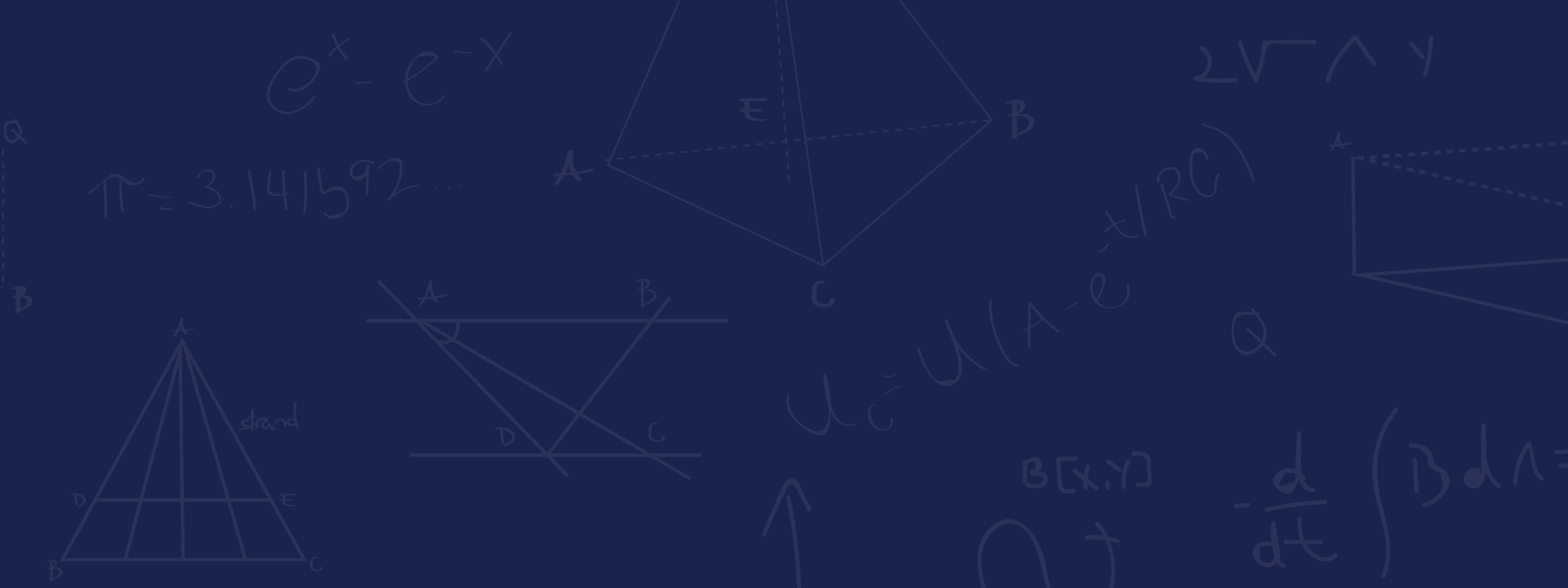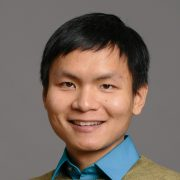# Greg Yang

Senior Researcher

I am currently developing a framework called Tensor Programs for understanding large (wide) neural networks, and more generally, computational graphs such as commonly seen in Pytorch/Tensorflow programs. This is the theoretical foundation that gave rise to the hyperparameter transfer paradigm for tuning enormous neural networks like GPT-3.

#### What is a Tensor Program (TP)?

Informally, a TP is just a composition of matrix multiplication and coordinatewise nonlinearities.

#### Why is it important?

It turns out that practically any computation in Deep Learning can be expressed as a TP, e.g. training a transformer on wikipedia data. Simultaneously, any Tensor Program has an “infinite-width” limit which can be derived from the program itself (through what’s called the Master Theorem). So this gives a universal way of taking the infinite-width limit of any deep learning computation, e.g. training an infinite-width transformer on wikipedia data.

Theoretically, this generalizes and unifies several lines of previous research, including the Neural Network-Gaussian Process Correspondence, Neural Tangent Kernel, mean field limit of shallow networks, and neural signal propagation, as well as areas outside of deep learning such as random matrix theory and approximate message passing. It also yields the maximal update parametrization and the feature learning limit (see below) for any neural architecture, which would have been hard to derive otherwise without the universality provided by the Tensor Programs machinery.

Practically, this maximal update parametrization (muP), for the first time, allows one to tune the hyperparameters of extremely large neural networks, too expensive to train more than once. This is because, in muP, narrow and wide neural networks share the same set of optimal hyperparameters. So one can tune the large model by just tuning a tiny version of it and copying over the hyperparameters.

#### How is this different from Neural Tangent Kernel (NTK)?

As mentioned above, NTK is a special case of TP. More importantly, a neural network in the NTK limit does not learn features — which contradicts the conventional wisdom that neural networks are successful because of its feature learning capability (e.g. conv nets and BERT). On the other hand, using TP, one can derive a limit that maximally learns features in a suitable sense (see TP4). When explicitly trained on tasks that depend crucially on feature learning (e.g. Word2Vec), this limit handily beats the NTK limit, as one would expect.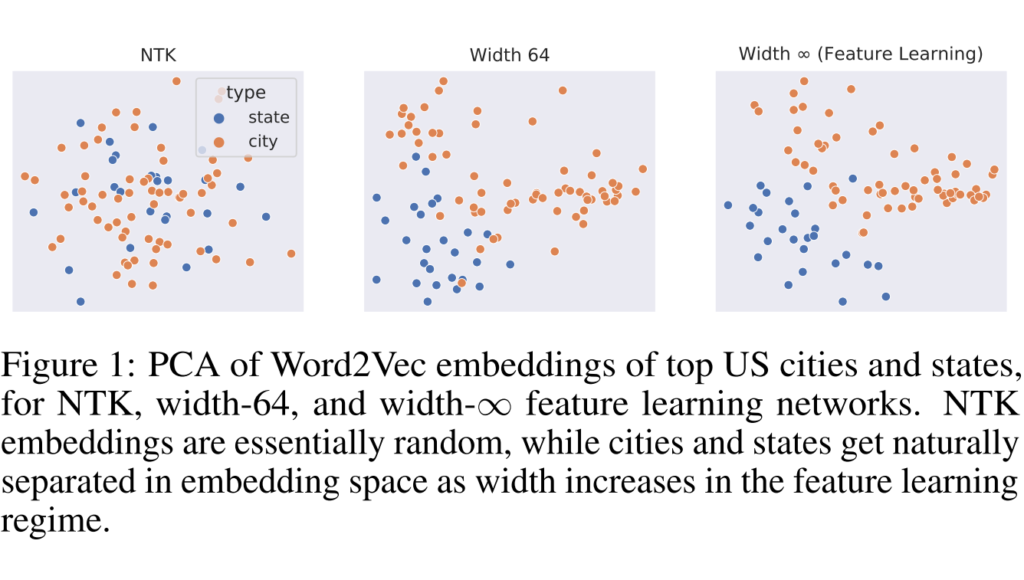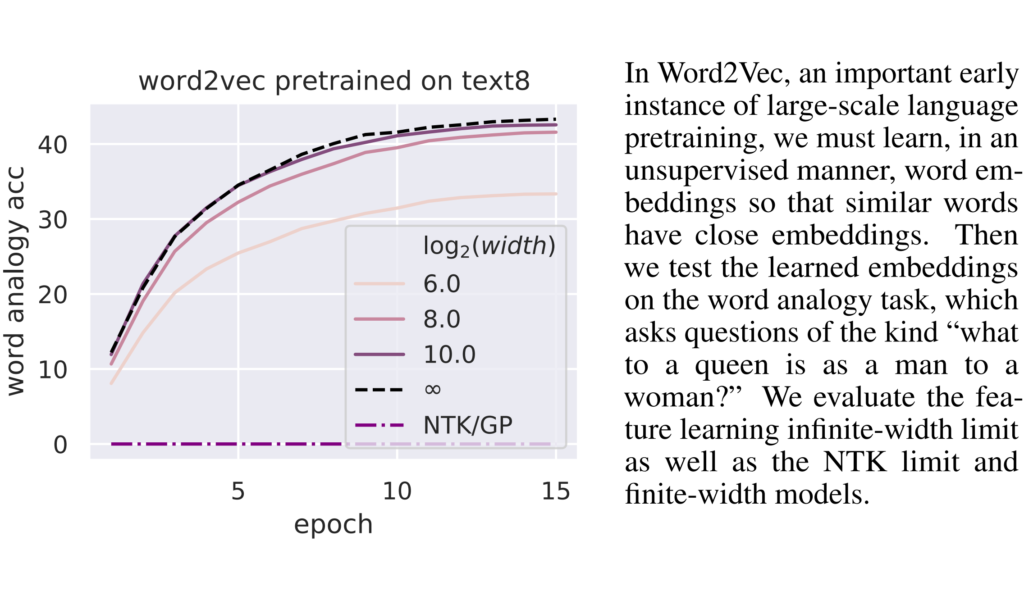#### Is there software to automatically convert my Pytorch/Tensorflow code to train infinite-width neural networks?

Working on it 🙂 In the mean time, if you don’t care about feature learning, then you can check out neural-tangents for kernel limits of common neural networks.

#### Summary of the papers on TP so far

• Arxiv

This is the original paper on Tensor Programs (so it could be named “Tensor Programs O”). It first established the paradigm of 1) using a formal language (Tensor Programs) to capture possible neural computations and 2) deriving an algorithm (the Master Theorem) for taking the infinite-width limit of any such program. All later papers will follow this paradigm.

Unfortunately for readers, the writing was very dense and notation cumbersome, so the next 3 papers focus more pedagogy and presentation of the material to a wider audience, as well as some minor but useful improvements of the results in this paper.

• This paper shows that an infinite-width neural network of any architecture (in the standard parametrization) are Gaussian processes at initialization. It also demonstrates how to calculate the kernel of this Gaussian process.

This is a consequence of the simplest form (called Netsor) of Tensor Programs, where one cannot use a matrix and its transpose in the same program, and its Master Theorem. Their theory is fully developed in a self-contained way here.

• This paper shows that the Neural Tangent Kernel of a neural network of any architecture (in the NTK parametrization) converges to a well-defined, deterministic kernel at initialization. It also demonstrates how to calculate the kernel. (Note it doesn’t yet say anything about training, which is done in Tensor Programs IIb briefed below).

This is a consequence of an extension of Netsor (called NetsorT), where one can use a matrix and its transpose in the same program in a restricted way. Its theory is fully developed in a self-contained way here.

• Arxiv

This paper continues from the above and shows that 1) the infinite-width Neural Tangent Kernel of a neural network of any architecture (in the NTK parametrization) stays frozen over the course of training, and 2) the infinite-width network evolves via kernel gradient descent with this kernel.

This paper actually needs the machinery of (and comes after) Tensor Programs III (described below), but we number this IIb because the topic is on NTK.

• Arxiv

This paper targets a slightly more mathematical audience than the previous papers. It shows how to calculate the Jacobian singular value distribution of an infinite-width neural network. As special cases, it recovers the classical semicircle and Marchenko-Pastur laws.

This is a consequence of the full version of Tensor Programs, where one can use a matrix and its transpose arbitrarily. Its theory is fully developed in a self-contained way here.

This paper is intended to serve as a rigorous reference for the TP foundation going forward.

• This paper derives “the” feature learning limit of neural networks, in contrast to the Neural Tangent Kernel limit, using machinery developed in the previous papers (especially TP3).

(Some trivia: this paper was supposed to be written right after TP0, but I realized the TP foundation needed to be solidified in terms of presentation and notation, hence TP1-3 and why this paper came almost two years later)

• You can’t train GPT-3 on a single GPU, much less tune its hyperparameters (HPs).

But what if I tell you…

…you *can* tune its HPs on a single GPU thanks to the theory developed in TP4?

Essentially, narrow and wide neural networks share the same set of optimal hyperparameters if they are in the maximal update parametrization (muP) derived in TP4 (but not if they are in pytorch default parametrization).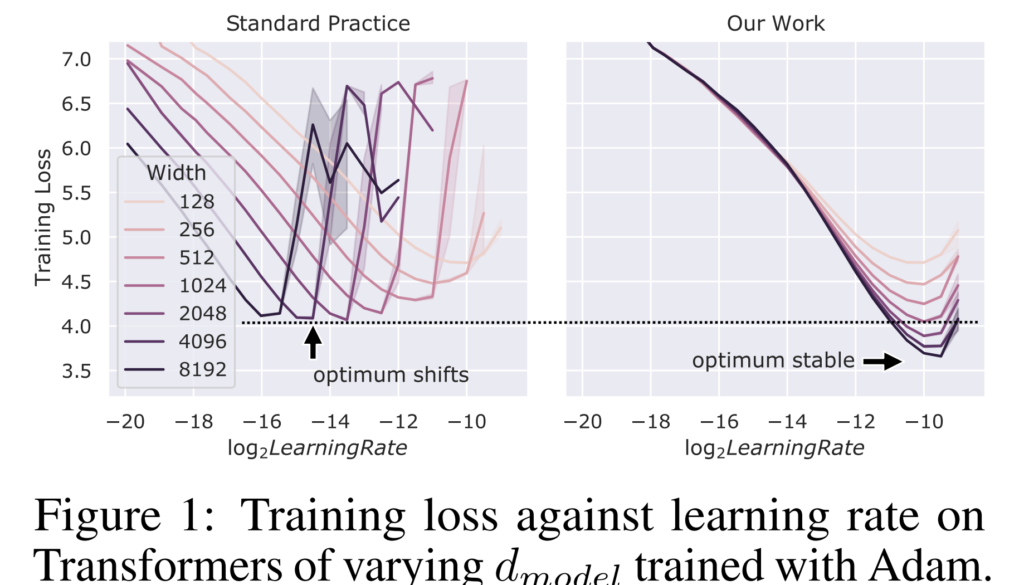This lets us transfer hyperparameters from small networks to large networks in the obvious way.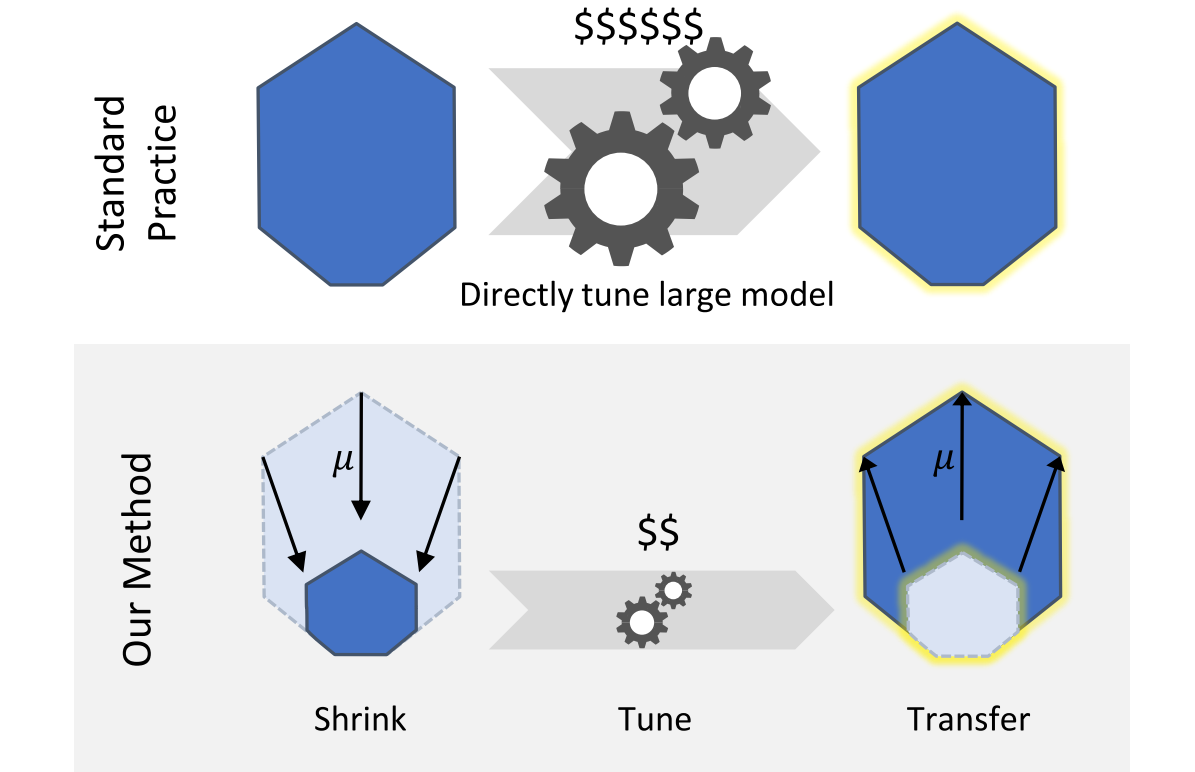The twitter thread above gives a nice but still brief overview of the key ideas and results.

(Some trivia: We finished most of the basic experiments in TP5 before I started writing TP2, at which my motivation for finish writing TP2-TP4 was just to provide enough foundation to write this paper)